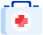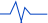[登录] [注册]| 服务热线：4008775957

A B C D F G H J K L M N P Q R S T W X Y Z

• A

• B

• C

• D

• F

• G

• H

• J

• K

• L

• M

• N

• P

• Q

• R

• S

• T

• W

• X

• Y

• Z

设计师谈单注意技巧

2021-11-29作者：企鹅公装网 被访问次数：92501建立专业形象02让客户信任03学会合理提问04倾听客户的需求05完善的沟通工具×

×